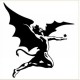# Is there any way to convert a number into string?

 -1 ``````char s1[] = "1996"; int a; a = atoi(s1); `````` / function atoi convert string into number but i want if b= 1000 then how it is stored in string s2[]; so that printf("%s",s2) will output 1000 / asked 23 Apr '16, 11:25SadhonRay 58●1●1●8

 0 There is a standard library function `itoa(int value, char *string, int base)` which converts integers into string. It takes three parameters. First parameter is the integer to convert, second parameter is the string which will hold the converted string and third parameter is the base in which the integer will be converted. ``````int main(){ char stringValue; int intValue = 1996; itoa(intValue, stringValue, 10); printf("Decimal: %s\n", stringValue); itoa(intValue, stringValue, 16); printf("Hexadecimal: %s\n", stringValue); itoa(intValue, stringValue, 2); printf("Binary: %s\n", stringValue); return 0; } `````` Output: ``````Decimal: 1996 Hexadecimal: 7cc Binary: 11111001011 `````` Note: Header file for itoa is `stdlib.h`. I can't write header files in the formatted code. Don't know why. Note: itoa isn't ANSI C Standard. So it won't compile in GCC under Linux. Here are some workarounds: itoa with GCC answered 23 Apr '16, 17:58Ashfaqur Rahman 783●9 Thanks.. I've understood.. (24 Apr '16, 14:50) SadhonRay If this helps you can upvote. If it answers your question you can choose this as correct answer, so that others can understand that this question contains an answer. (24 Apr '16, 14:53) Ashfaqur Rahman
 toggle preview community wiki:

### Follow this question

By Email:

Once you sign in you will be able to subscribe for any updates here

Markdown Basics

• *italic* or _italic_
• **bold** or __bold__
• image?![alt text](/path/img.jpg "title")
• numbered list: 1. Foo 2. Bar
• to add a line break simply add two spaces to where you would like the new line to be.
• basic HTML tags are also supported

Question tags:

×402
×56

question asked: 23 Apr '16, 11:25

question was seen: 636 times

last updated: 24 Apr '16, 14:53